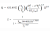HOT

# Natural Gas Pipeline Flow Calculations

\$149.00

Format On-demand 2 hrs Intermediate Yes 1 Year 24/7 English Dr. Harlan Bengtson

## What you will learn

• Familiarity with the natural gas properties, density, specific gravity, molecular weight, compressibility factor, and viscosity, and their use in pipeline flow calculations.
• Calculate the compressibility factor for natural gas with specified average gas pressure and temperature and known specific gravity.
• Calculate the viscosity of natural gas with specified average gas pressure and temperature and known specific gravity.
• Obtain a value for the friction factor using the Moody diagram for given Re and e/D.
• Calculate a value for the friction factor for specified Re and e/D, using the appropriate equation for f.
• Use the Panhandle A equation to calculate gas flow rate through a pipe with known diameter and length, elevation difference between pipeline inlet and outlet, specified inlet and outlet pressure and enough information to calculate gas properties.
• Familiarity with the guidelines for when it is appropriate to use the Darcy Weisbach equation for natural gas pipeline flow calculations.
• Be able to use the Darcy Weisbach equation and the Moody friction factor equations to calculate the frictional pressure drop for a given flow rate of a specified fluid through a pipe with known diameter, length and roughness.
• Be able to use the Weymouth equation to calculate gas flow rate through a pipe with known diameter and length, elevation difference between pipeline inlet and outlet, specified inlet and outlet pressure and enough information to calculate gas properties.
• Be able to use the Panhandle B equation to calculate gas flow rate through a pipe with known diameter and length, elevation difference between pipeline inlet and outlet, specified inlet and outlet pressure and enough information to calculate gas properties.

## Description

Several different equations have been proposed and are in use for natural gas pipeline flow calculations. This course provides information about four of them. More specifically we will cover:

• Natural Gas Properties
• Laminar and Turbulent Flow in Pipes
• Options for Natural Gas Pipeline Calculations
• The Darcy Weisbach Equation
• The Weymouth Equation
• The Panhandle A Equation
• The Panhandle B Equation
• Examples Calculations
• Summary
• References

## Curriculum

• Natural Gas Properties
• Laminar and Turbulent Flow in Pipes
• Options for Natural Gas Pipeline Calculations
• The Darcy Weisbach Equation
• The Weymouth Equation
• The Panhandle A Equation
• The Panhandle B Equation
• Examples Calculations
• Summary
• References

## Target Audience

• Petroleum engineers,
• Chemical engineers,
• Geotechnical engineers,
• Environmental engineers, and
• Civil engineers
An attendee of this course will gain knowledge about four equations commonly used for natural gas pipeline flow calculations and how to use them.

## Specification: Natural Gas Pipeline Flow Calculations

Format On-demand 2 hrs Intermediate Yes 1 Year 24/7 English Dr. Harlan Bengtson

## About the Publisher

•• Store Name: Harlan
• Dr. Bengtson is a Professional Engineer with 30 years experience as an engineering professor, teaching fluid mechanics, thermodynamics and a variety of other mechanical and civil engineering courses. ...   Read More >

## Reviews

##### 0.0
0
0
0
0
0

There are no reviews yet.Natural Gas Pipeline Flow Calculations

\$149.00

\$149.00

30-day money back guarantee
1-Year access
Secure encrypted payment
All Credit cards AcceptedSKU: PL 876950 Categories: ,
Format On-demand 2 hrs Intermediate Yes 1 Year 24/7 English Dr. Harlan Bengtson

Enter your account data and we will send you a link to reset your password.

## Your password reset link appears to be invalid or expired.